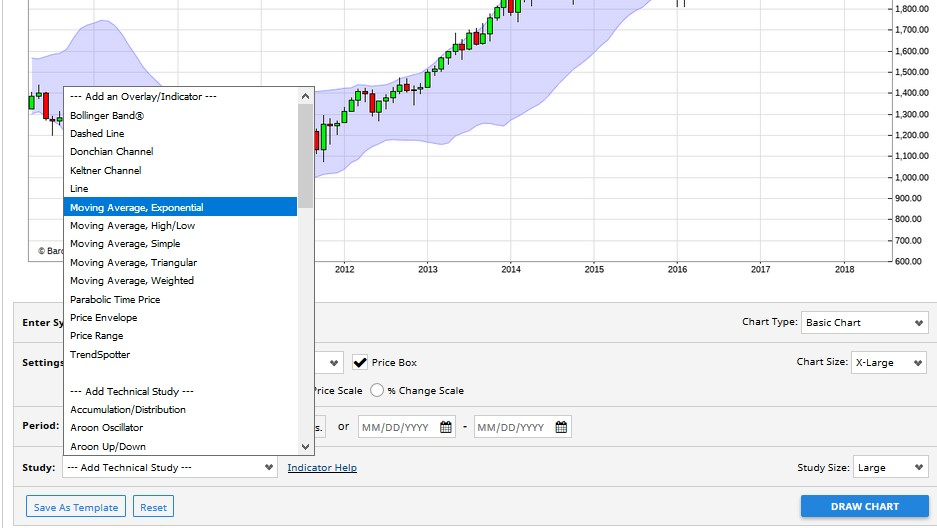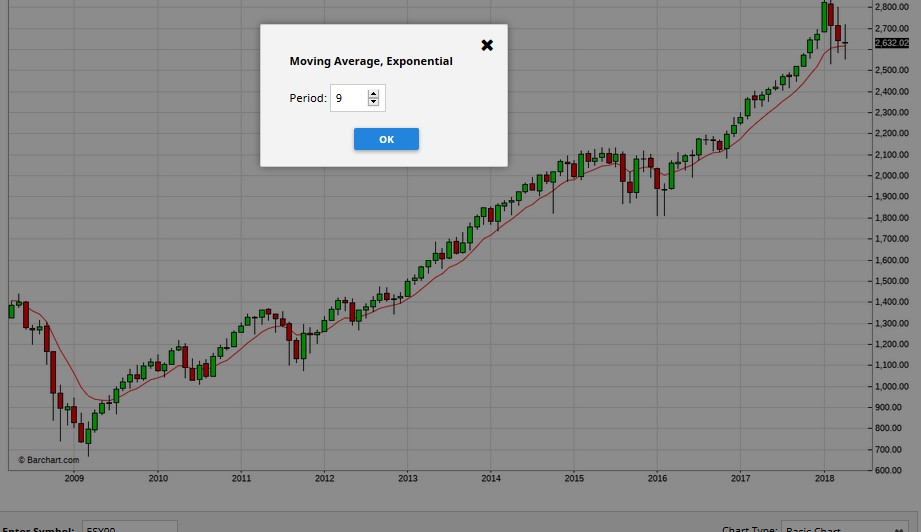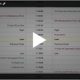# Understanding Moving Averages

Futures Education Homepage

Exponential Moving Average (Red Line)
xXx
1) To Set a Exponential Moving Average
open this chart  (or 6-9 to calculate)
xxx
x

3) Choose Moving Average Exponential
x

x4) Click on the default parameter
x5) Set the desired number of days
xMoving averages are a common way for technical traders to begin the process of price analysis. It is often one of the first indicators that traders will add to their charts and will serve as a measure on its own or in comparison with other indicators.

A moving average is the average price of a futures contract or stock over a set period of time. Traders can add just one moving average or have many different time frames on one chart.

For example, a 14-day moving average of CL WTI futures would be the average closing price of the CL contract over the last 14 days.

7) Calculating Moving Average

There are a number of ways to mathematically calculate the average of a set of numbers. Each method will come up with a slightly different result and place emphasis on a certain section of the data being calculated.

Two common moving average calculations are simple moving averages and exponential moving averages. These moving averages will appear on a chart as a line above or below price. Traders might have multiple moving averages on their charts at one time and use different lines to represent different actions you might take with your trades

8) Simple Moving Average

A simple moving average, the most basic of moving averages, is calculated by summing up the closing prices of the last x days and dividing by the number of days.

For example, if WTI (CL) contract closed at \$45.50, \$45.25 and \$46.10 over the last three days the moving average would be calculated as follows:

```Sum of closing prices = 45.50 + 45.25 + 46.10 = 136.85
Simple moving average = sum of closing prices divided by number of days
= 136.85 / 3
= \$45.62```

9) Exponential Moving Averages

Exponential moving averages assign more influence on recent numbers and less on old data because of a weighting variable in the calculation. This makes them more responsive to changes in price and also acts in smoothing out the line.

Exponential moving averages calculate the average of a series of numbers using a weighting multiplier that typically assigns more weight to later data. EMAs can be calculated in three steps.

1. Determine the SMA or use yesterday’s closing price to begin

2. Calculate the multiplier

3. Using price, the multiplier (time period) and the previous EMA value.

Here is the calculation for a 14-day EMA

```1. SMA = \$46.60, Closing price today is \$46.75
2. Multiplier = 2 / (1 + n) = 2 / ( 1 + 14) = 0.133
3. Calculate the EMA = (Price today x Multiplier) + (EMA yesterday x ( 1 – multiplier)
EMA = (46.75 x 0.133) + (46.60 x 0.867)
EMA = \$46.63```

Note the first day of the EMA calculation can either start with yesterday’s closing price or the SMA from yesterday. You just need to pick a starting value for the EMA calculation.

As with simple moving averages, no calculation is needed on your part, the moving average indicator will calculate this for you and show the results as a line on your chart.

While there are other more complicated moving average calculations beyond EMA and SMA, these two are the most common. Other moving averages are basically an EMA that assigns different weighting and smoothing variables to the calculations.

10) Using Moving Averages

Moving averages are often used to compare where the current price of the underlying instrument is in relation to support and resistance on a chart. When price moves down to a moving average line or up to a moving average line, traders can use this as a signal that price might stop or retrace at that point.

For example, if price moved down to the 200EMA a trader might think that price might stop moving down from there as the 200 EMA will act as support for price to move back up.

Traders can also visualize short-term and long-term support and resistance on a chart by adding moving average lines of different time periods.

For example, a trader could use the 13EMA as a short-term indicator and the 200 EMA as a longer-term indicator on the same chart. The larger the EMA, the stronger the support and resistance and the more likely the price will change direction as it moves towards that EMA.

Of particular interest for traders can be when moving averages cross over, as these crossovers usually represent a shift in price. Crossovers, which occur when one moving average line crosses another moving average line, is used to signal bullish and bearish signals.

Short-term moving averages crossing above longer-term moving averages is generally seen as bullish and long-term moving averages crossing below shot-term moving averages is generally seen as bearish.

For example, if a trader sees that the 50 EMA is crossing above the 200 EMA this is generally a sign that price might continue to move up. A trader using moving averages as a signal to enter trades might purchase contracts, or add to a position because of this crossover signal.

Moving averages are simple yet powerful tools that traders can use to help visualize where price has been and where price might be moving next.

If you have questions send us a message or schedule an online review.

Regards,

—————————————————————-

Privacy Notice

Disclosure